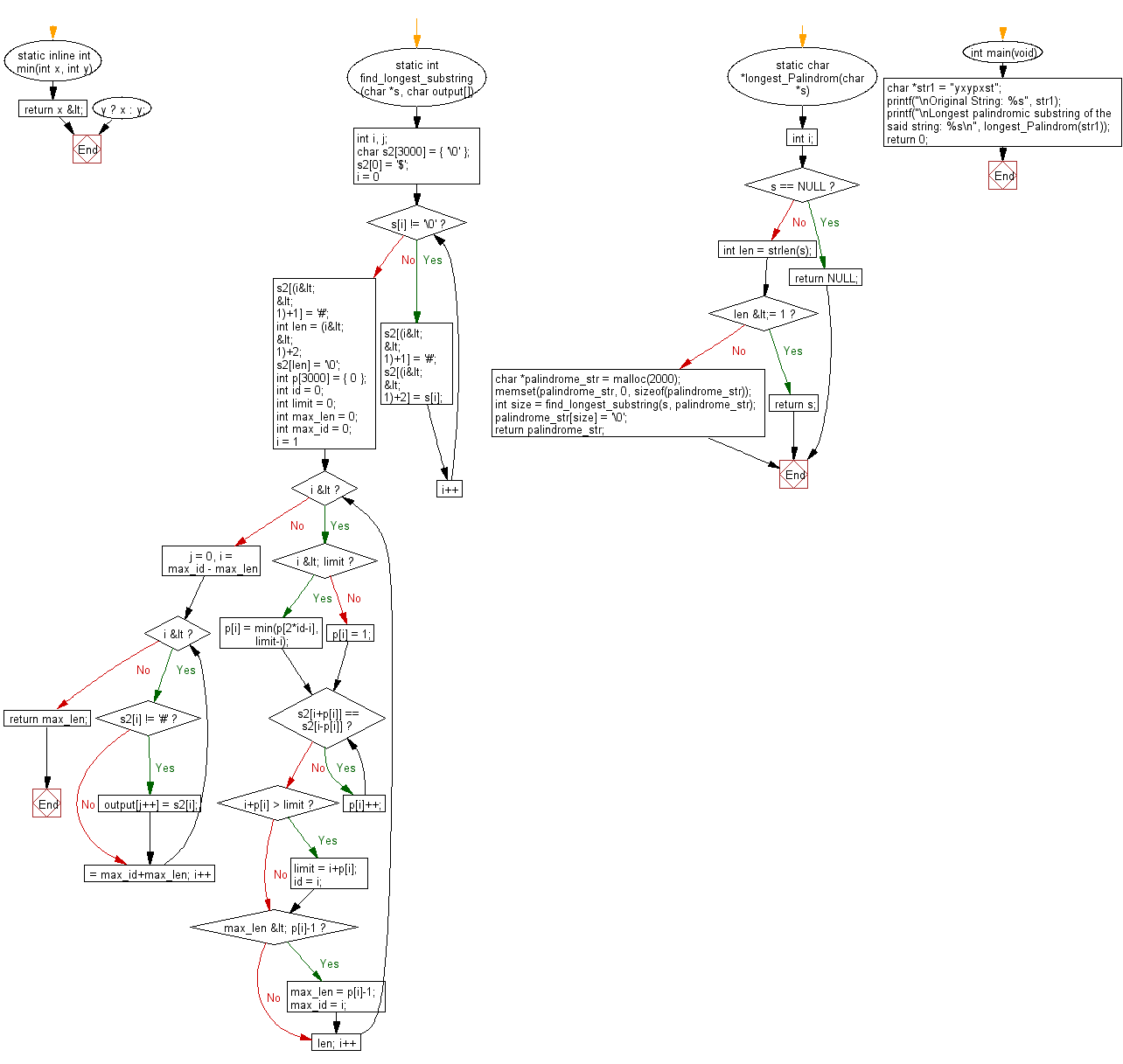﻿ C Program: Longest palindromic substring of a string# C Exercises: Find the longest palindromic substring of a given string

## C Programming Practice: Exercise-4 with Solution

Write a C programming to find the longest palindromic substring of a given string.Maximum length of the given string is 1000.

C Code:

``````#include <stdio.h>
#include <stdlib.h>
#include <string.h>

static inline int min(int x, int y)
{
return x < y ? x : y;
}
static int find_longest_substring(char *s, char output[])
{
int i, j;
char s2 = { '\0' };

s2 = '\$';
for (i = 0; s[i] != '\0'; i++) {
s2[(i<<1)+1] = '#';
s2[(i<<1)+2] = s[i];
}
s2[(i<<1)+1] = '#';
int len = (i<<1)+2;
s2[len] = '\0';

int p = { 0 };
int id = 0;
int limit = 0;
int max_len = 0;
int max_id = 0;
for (i = 1; i < len; i++) {
if (i < limit) {
p[i] = min(p[2*id-i], limit-i);
} else {
p[i] = 1;
}

while (s2[i+p[i]] == s2[i-p[i]]) {
p[i]++;
}

if (i+p[i] > limit) {
limit = i+p[i];
id = i;
}
if (max_len < p[i]-1) {
max_len = p[i]-1;
max_id = i;
}
}

for (j = 0, i = max_id - max_len; i <= max_id+max_len; i++) {
if (s2[i] != '#') {
output[j++] = s2[i];
}
}
return max_len;
}

static char *longest_Palindrom(char *s)
{
int i;
if (s == NULL) {
return NULL;
}

int len = strlen(s);
if (len <= 1) {
return s;
}

char *palindrome_str = malloc(2000);
memset(palindrome_str, 0, sizeof(palindrome_str));

int size = find_longest_substring(s, palindrome_str);
palindrome_str[size] = '\0';
return palindrome_str;
}

int main(void)
{
char *str1 = "yxypxst";
printf("\nOriginal String: %s", str1);
printf("\nLongest palindromic substring of the said string: %s\n", longest_Palindrom(str1));
return 0;
}

``````

Sample Output:

```Original String: yxypxst
Longest palindromic substring of the said string: yxy
```

Pictorial Presentation:Flowchart:## C Programming Code Editor:

What is the difficulty level of this exercise?

Test your Programming skills with w3resource's quiz.

﻿

## C Programming: Tips of the Day

Maximum value of int:

In C:

```#include <limits.h>
then use
int imin = INT_MIN; // minimum value
int imax = INT_MAX;```

or

```#include <float.h>

float fmin = FLT_MIN;  // minimum positive value
double dmin = DBL_MIN; // minimum positive value

float fmax = FLT_MAX;
double dmax = DBL_MAX;```

Ref : https://bit.ly/3fi8yk9